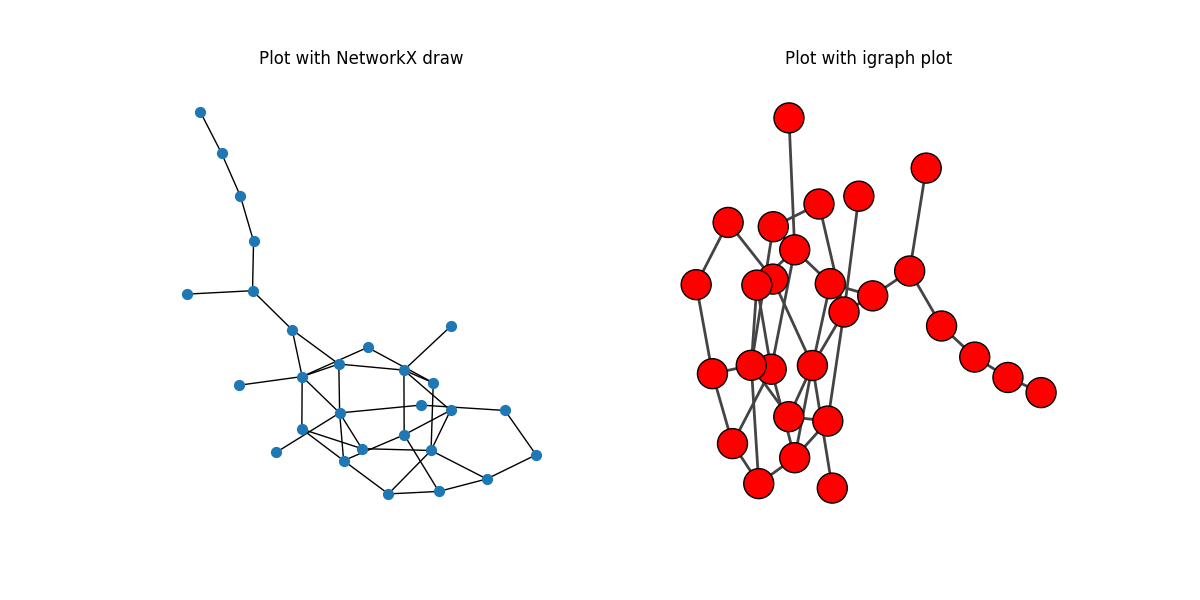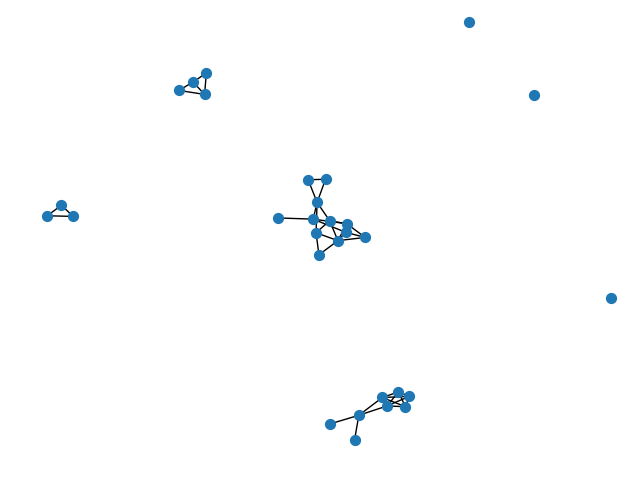# igraph#

igraph (https://igraph.org/) is a popular network analysis package that provides (among many other things) functions to convert to/from NetworkX.

```import matplotlib.pyplot as plt
import networkx as nx
import igraph as ig
```

## NetworkX to igraph#

```G = nx.dense_gnm_random_graph(30, 40, seed=42)

# largest connected component
components = nx.connected_components(G)
largest_component = max(components, key=len)
H = G.subgraph(largest_component)

# convert to igraph
h = ig.Graph.from_networkx(H)

# Plot the same network with NetworkX and igraph
fig, (ax0, ax1) = plt.subplots(nrows=1, ncols=2, figsize=(12, 6))

# NetworkX draw
ax0.set_title("Plot with NetworkX draw")

# igraph draw
ax1.set_title("Plot with igraph plot")
ig.plot(h, layout=layout, target=ax1)
plt.axis("off")
plt.show()
```## igraph to NetworkX#

```g = ig.Graph.GRG(30, 0.2)
G = g.to_networkx()
nx.draw(G, node_size=50)
plt.show()
```Total running time of the script: ( 0 minutes 0.361 seconds)

Gallery generated by Sphinx-Gallery